##### Trigonometry Workbook For DummiesWith the trigonometric substitution method, you can do integrals containing radicals of certain forms because they match up with trigonometric functions. A sine can take the place of a radical in a particular form.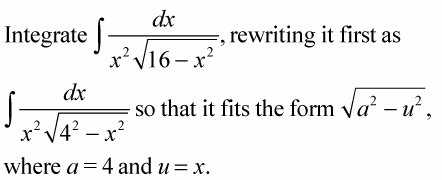1. Draw a right triangle where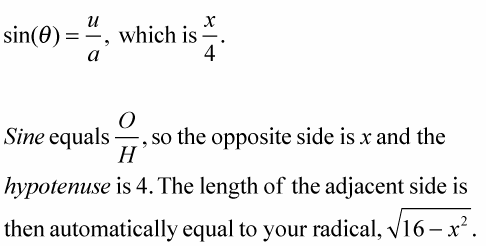You should confirm this with the Pythagorean theorem.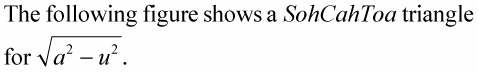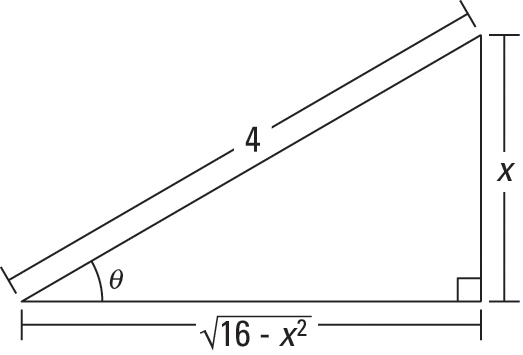2. Solve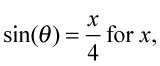differentiate, and solve for dx.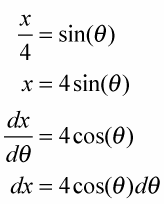3. Find which trig function equals the radical over the a, and then solve for the radical.

Look at the triangle in the figure.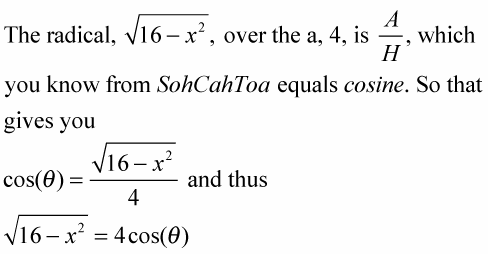4. Use the results from Steps 2 and 3 to make substitutions in the original problem and then integrate.

Note that in this particular problem, you have to make three substitutions, not just two like in the first example.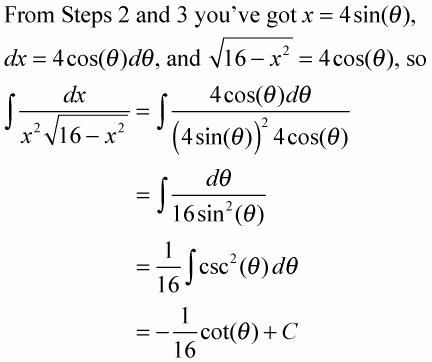5. The triangle shows that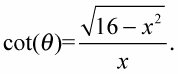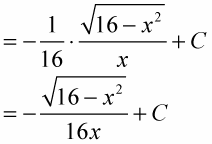It’s a walk in the park.